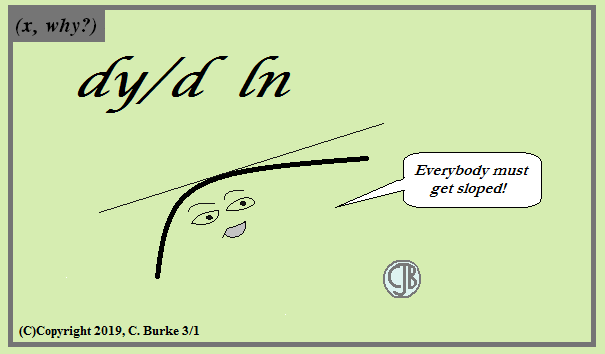## Saturday, March 30, 2019

### Vertical Angles

(Click on the comic if you can't see the full image.)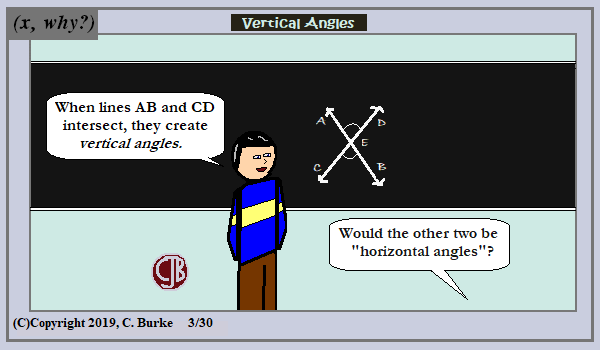I've been asked, and it's not unreasonable if they don't understand (or remember) where the word "vertical" comes from.

Come back often for more funny math and geeky comics.## Monday, March 25, 2019

### Foregone Conclusion

(Click on the comic if you can't see the full image.)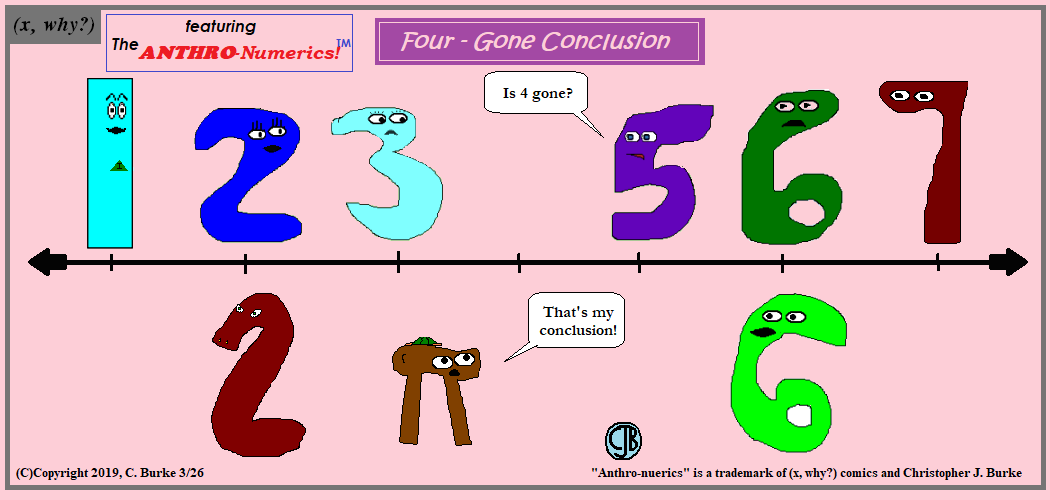You know he won't be gone 4 long!

You may have notice my "new" name for my talking numerics. Except it isn't really a new name -- to me. I came up with the name back in 2007, when the strip was new, but I was afraid to use it because I thought someone would steal it from me. And I was a nobody at the time. I figured I needed to register my trademark, but I never got around to doing that (or even figuring out exactly how). By my tenth anniversary, I was wondering what the hell am I waiting for? I had a great name but no one else knew about it.

So I hope you like it, and I'll keep on using it. Now that I've a bit more established, I'll use it more frequently. I don't think I'll retcon the old strips, but if I ever update the Archives, I'll be sure to add a note.

Come back often for more funny math and geeky comics.## Friday, March 22, 2019

### When Will We Use This?

(Click on the comic if you can't see the full image.)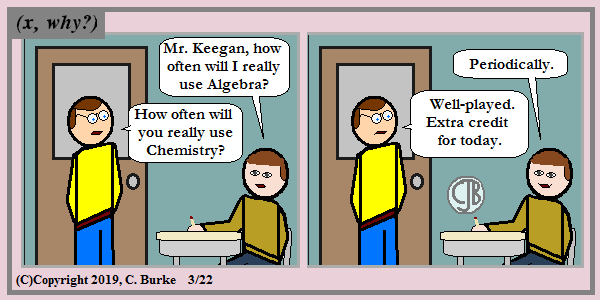He could've said History, but they're doomed to repeat it.

Come back often for more funny math and geeky comics.## Thursday, March 14, 2019

### The Casebook of Sherlock Pi: The Adventure of the Missing Three-Quarters

(Click on the comic if you can't see the full image.)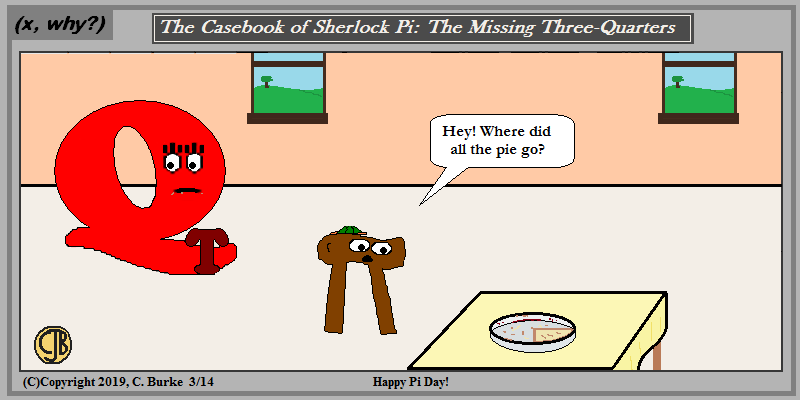There was one clear suspect.
I thought about going with a piggy bank and spare change, but you know what today is, right?

Happy Pi Day!

Come back often for more funny math and geeky comics.## Tuesday, March 12, 2019

### January 2019 Common Core Geometry Regents, Parts 3 and 4 (open-ended)

The following are some of the multiple questions from the recent January 2019 New York State Geometry Regents exam.
The questions and answers to Part I can be found here.
The questions and answers to Part II can be found here.

### January 2019 Geometry, Part III

Each correct answer is worth up to 4 credits. Partial credit is available. Work must be shown. Correct answers without work receive only 1 point.

32. A triangle has vertices A(-2,4), B(6,2), and C(l, -1).
Prove that 6.ABC is an isosceles right triangle. [The use of the set of axes below is optional.]

The grid isn't necessary, but quite helpful. Plot the points, and draw and label the triangle.
To prove that ABC is an isosceles right triangle, you have to show two things: that it's right and that it's isosceles. Do not leave either part out.
To prove that it's right, you need to show that two of the sides have perpendicular slopes.
To prove that it's isosceles, you have to show that the two legs are the same length. Note that if you show that it's a right triangle first, you don't have to bother with the hypotenuse, which is the longest side.
So this is a good reason for sketching the triangle: It will be "obvious" which angle is the right angle. That isn't enough, and you can't state that, but you can use that fact to test the two legs first.

From the drawing, you can see that if ABC is a right triangle, C is the right angle and AC = BC. This is what we need to test.
Also, by graphing, you can do all the work WITHOUT using any formulas.
Slope of AC = -5/3 (5 down, 3 right). Slope of BC = 3/5 (3 up, 5 right). Since (-5/3)(3/5) = -1, the lines are perpendicular. (The slopes are inverse reciprocals.) So there is a right angle.
Now draw two right triangles (if you didn't when calculating slopes) with AC and BC as hypotenuses.
Label the legs 5 and 3, and mark the right angle. By SAS, these two triangles are congruent and therefore AC is congruent to BC.
Or using Pythagorean Theorem, 52 + 32 = AC2 and 52 + 32 = BC2, so AC = BC.
So ABC is a right triangle and ABC is isosceles.

If you didn't draw the triangle, you need to use the formulas to show all the work.
Slope of AB = (4 - 2) / (-2 - 6) = 2 / -8 = -1/4
Slope of AC = (-1 - 4) / (1 - -2) = -5 / 3
Slope of BC = (-1 - 2) / (1 - 6) = -3 / -5 = 3/5
AC and BC are negative reciprocals, so AC is perpendicular to BC, which creates a right angle.
Legs AC and BC must be equal, so use the Distance Formula.
SQR ((1 - -2)2 + (-1 - 4)2) = SQRT (9 + 25) = SQRT(34)
SQR ((1 - 6)2 + (-1 - 2)2) = SQRT (25 + 9) = SQRT(34)
AC = BC, so the triangle is isosceles.

33. Theresa has a rectangular pool 30 ft long, 15 ft wide, and 4 ft deep. Theresa fills her pool using city water at a rate of \$3.95 per 100 gallons of water.
Nancy has a circular pool with a diameter of 24 ft and a depth of 4 ft. Nancy fills her pool with a water delivery service at a rate of \$200 per 6000 gallons.
If Theresa and Nancy both fill their pools 6 inches from the top of the pool, determine and state who paid more to fill her pool. [ 1 ft3 water = 7.48 gallons]

You need to find the Volume of both pools, minus six inches, which is .5 feet. And then you need to calculate the cost to fill the pools. It doesn't say, but I'm going to calculate based on pro rating the cost, so Nancy isn't paying \$200 or \$400 or \$600, like you might pay if you go over on your data limits on your phone.
Remember to use the radius, which is 24/2 = 12, and not the diameter.

VT = L * W * H = 30 * 15 * 3.5 = 1575 and 1575 * 7.48 = 11,781
CostT = 1575 * 3.95 / 100 = 465.3495 = \$465.35
VN = (pi)r2h = (3.141592)(12)2(3.5) = 1583.362368, and 1583.362368 * 7.48 = 11843.5505126...
CostN = 11843.5505126 * 200 / 6000 = 394.785017087 = \$394.78
Theresa paid more to fill her pool than Nancy did.

34. As modeled in the diagram below, an access ramp starts on flat ground and ends at the beginning of the top step. Each step is 6 inches tall and 8 inches deep.

If the angle of elevation of the ramp is 4.76°, determine and state the length of the ramp, to the nearest tenth of a foot.
Determine and state, to the nearest tenth of a foot, the horizontal distance, d, from the bottom of the stairs to the bottom of the ramp

First part: the angle of elevation is 4.76°, and the height (the opposite side) is 18 inches, the sum of the three sixes in the diagram. But the answer is needed in feet, so you need to convert.
18 inches * (1 foot / 12 inches) = 1.5 feet.
Sin 4.76 = 1.5 / x
x = 1.5 / sin 4.76 = 18.076... = 18.1 feet
Second part: Note that d does not extend to the end of the right triangle, it stops 16 inches before it. Whatever answer you get, you need to subtract 16 inches from it (or 1.3333.... feet).
You can use cosine or Pythagorean theorem at this point. I'll show both.

cos 4.76 = y / 18.1
y = 18.1 * cos 4.76 = 18.037
d = y - 1.333 = 18.037 - 1.333 = 16.704 = 16.7 feet.

1.52 + y2 = 18.12
2.25 + y2 = 327.61
y2 = 327.61 - 2.25 = 325.36 = 18.038
d = 18.038 - 1.333 = 16.705 = 16.7 feet.

Note that there's an error caused by rounding after at the third decimal place. However, since we are rounding to the nearest tenth, the difference is not significant.

### Part IV

A correct answer is worth up to 6 credits. Partial credit is available.

35. In the diagram of quadrilateral ABCD with diagonal shown below, segments GH and EF are drawn, AE = CG, BE = DG, AH = CF, and AD = CB.

Prove: EF = GH

Strategy: First of all, note that the figure is a "quadrilateral". You are not given that it is a "parallelogram", so you cannot use properties of parallelograms unless you first prove that ABCD is a parallelogram. You don't need to do that, but you do need to show that the two larger triangles (ABC and ADC) are congruent. This will give you the angle that the smaller triangles share. Using the addition postulate, you can show that AF = CH, which will prove that the two smaller triangles are congruent. Finally, we'll use CPCTC (Corresponding Parts of Congruent Triangles are Congruent, or "Definition of Congruent Polygons") to show that EF = GH.

 Statements Reasons 1. Quadrilateral ABCD with diagonal AC, GH and EF, AE = CG, BE = DG, AH = CF, HD = CB Given 2. AC = AC Reflexive 3. AE + BE = GC + DG Addition Postulate 4. Triangle ABC = Triangle CDA SSS Postulate 5. Angle EAF = Angle GCH CPCTC 6. HF = HF Reflexive Property 7. AH + HF = FC + HF Addition Postulate 8. Triangle AEF = Triangle CGH SAS 9. EF = GH CPCTC

End of Exam

How did you do?
Comments and corrections welcome. (I get many of the latter!)

## Monday, March 11, 2019

### Give Up For Lent

(Click on the comic if you can't see the full image.)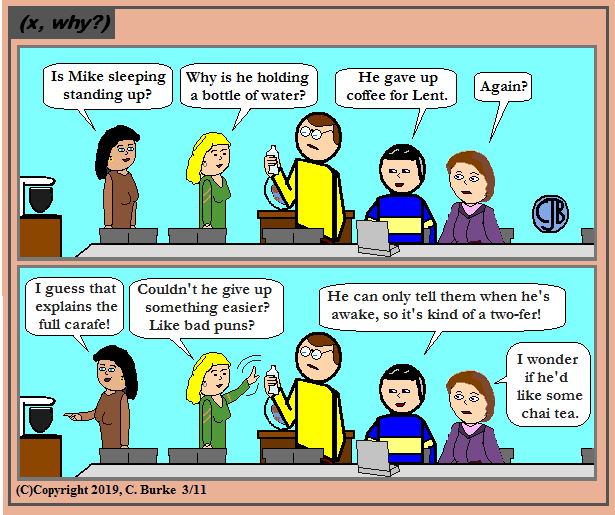This was supposed to be Friday's comic, but I was too tired. Bit of a headache, too.

(Please don't reply "I gave up giving up things for Lent." That was old in the 80s. Thank you.)

Come back often for more funny math and geeky comics.## Friday, March 01, 2019

### Sloped

(Click on the comic if you can't see the full image.)